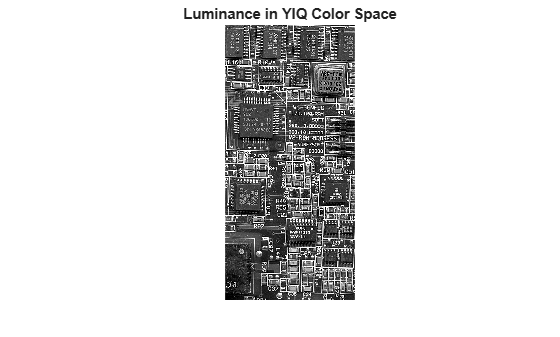# rgb2ntsc

Convert RGB color values to NTSC color space

## Syntax

``YIQ = rgb2ntsc(RGB)``

## Description

example

````YIQ = rgb2ntsc(RGB)` converts the red, green, and blue values of an RGB image to luminance (Y) and chrominance (I and Q) values of an NTSC image.```

## Examples

collapse all

This example shows how to convert an image from RGB to NTSC color space.

Read an RGB image into the workspace.

`RGB = imread('board.tif');`

Convert the image to YIQ color space.

`YIQ = rgb2ntsc(RGB);`

Display the NTSC luminance value, represented by the first color channel in the YIQ image.

```imshow(YIQ(:,:,1)); title('Luminance in YIQ Color Space');```## Input Arguments

collapse all

RGB color values to convert, specified as a numeric array in one of these formats.

• c-by-3 colormap. Each row specifies one RGB color value.

• m-by-n-by-3 image

Data Types: `single` | `double` | `uint8` | `uint16` | `int16`

## Output Arguments

collapse all

Converted YIQ color values, returned as a numeric array of the same size as the input.

AttributeDescription
YLuma, or brightness of the image. Values are in the range [0, 1], where 0 specifies black and 1 specifies white. Colors increase in brightness as Y increases.
IIn-phase, which is approximately the amount of blue or orange tones in the image. I in the range [-0.5959, 0.5959], where negative numbers indicate blue tones and positive numbers indicate orange tones. As the magnitude of I increases, the saturation of the color increases.
QQuadrature, which is approximately the amount of green or purple tones in the image. Q in the range [-0.5229, 0.5229], where negative numbers indicate green tones and positive numbers indicate purple tones. As the magnitude of Q increases, the saturation of the color increases.

Data Types: `double`

## Algorithms

In the NTSC color space, the luminance is the grayscale signal used to display pictures on monochrome (black and white) televisions. The other components carry the hue and saturation information. The value `0` corresponds to the absence of the component, while the value `1` corresponds to full saturation of the component.

`rgb2ntsc` defines the NTSC components using

`$\left[\begin{array}{c}Y\\ I\\ Q\end{array}\right]=\left[\begin{array}{rrr}\hfill 0.299& \hfill 0.587& \hfill 0.114\\ \hfill 0.596& \hfill -0.274& \hfill -0.322\\ \hfill 0.211& \hfill -0.523& \hfill 0.312\end{array}\right]\left[\begin{array}{c}R\\ G\\ B\end{array}\right]$`